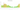# £

## Saint Helena pound

### St. Helena pound

#### how has the value of the currency changed in the last year?## USD 1 = SHP 0.81536

How much is10 US dollars worth inSt. Helena pounds?
At the current exchange rate, 10 US dollars is worth 8.15 St. Helena pounds
How much is50 US dollars worth inSt. Helena pounds?
At the current exchange rate, 50 US dollars is worth 40.77 St. Helena pounds
How much is100 US dollars worth inSt. Helena pounds?
At the current exchange rate, 100 US dollars is worth 81.54 St. Helena pounds
How much is500 US dollars worth inSt. Helena pounds?
At the current exchange rate, 500 US dollars is worth 407.68 St. Helena pounds
How much is2,000 US dollars worth inSt. Helena pounds?
At the current exchange rate, 2,000 US dollars is worth 1,630.72 St. Helena pounds
How much is10 US dollars worth inSt. Helena pounds?
At the current exchange rate, 10 US dollars is worth 8.15 St. Helena pounds
How much is50 US dollars worth inSt. Helena pounds?
At the current exchange rate, 50 US dollars is worth 40.77 St. Helena pounds
How much is100 US dollars worth inSt. Helena pounds?
At the current exchange rate, 100 US dollars is worth 81.54 St. Helena pounds
How much is500 US dollars worth inSt. Helena pounds?
At the current exchange rate, 500 US dollars is worth 407.68 St. Helena pounds
How much is2,000 US dollars worth inSt. Helena pounds?
At the current exchange rate, 2,000 US dollars is worth 1,630.72 St. Helena pounds